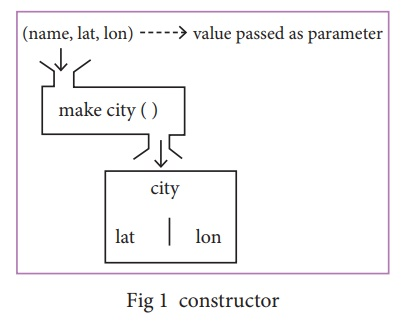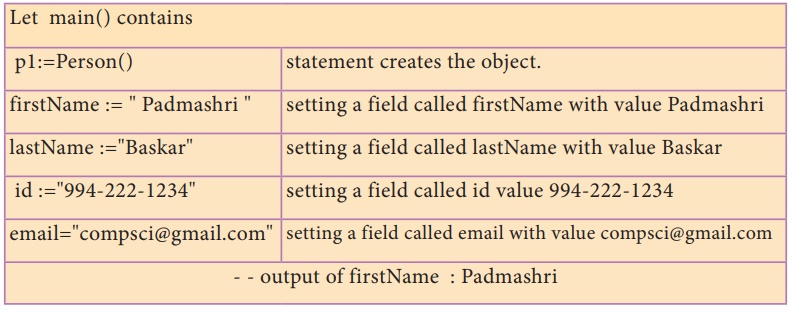Home | | Computer Science 12th Std | Data Abstraction: Book Back Questions and Answers

# Data Abstraction: Book Back Questions and Answers

Computer Science : Data Abstraction

Evaluation

Part – I

## Choose the best answer (1 Mark)

1. Which of the following functions that build the abstract data type ?

(A) Constructors

(B) Destructors

(C) recursive

(D)Nested

2.  Which of the following functions that retrieve information from the data type?

(A) Constructors

(B) Selectors

(C) recursive

(D)Nested

3.  The data structure which is a mutable ordered sequence of elements is called

(A) Built in

(B) List

(C) Tuple

(D) Derived data

4.  A sequence of immutable objects is called

(A) Built in

(B) List

(C) Tuple

(D) Derived data

5.  The data type whose representation is known are called

(A) Built in datatype

(B) Derived datatype

(C) Concrete datatype

(D) Abstract datatype

6.  The data type whose representation is unknown are called

(A) Built in datatype

(B) Derived datatype

(C) Concrete datatype

(D) Abstract datatype

7.  Which of the following is a compound structure?

(A) Pair

(B) Triplet

(C) single

8.  Bundling two values together into one can be considered as

(A) Pair

(B) Triplet

(C) single

9.  Which of the following allow to name the various parts of a multi-item object?

(A) Tuples

(B) Lists

(C) Classes

10.     Which of the following is constructed by placing expressions within square brackets?

(A) Tuples

(B) Lists

(C) Classes

Part – II

## Answer the following questions (2 Marks)

1. What is abstract data type?

Ans. (i) Abstract Data type (ADT) is a type (or class) for objects whose behavior is defined by a set of value and a set of operations.

(ii) The definition of ADT only mentions what operations are to be performed but not how these operations will be implemented.

(iii) It does not specify how data will be organized in memory and what algorithms will be used for implementing the operations. It is called “abstract” because it gives an implementation independent view. The process of providing only the essentials and hiding the details is known as abstraction.

2. Differentiate constructors and selectors.

Ans. (i) Constructors are functions that build the abstract data type.

(ii) Selectors are functions that retrieve information from the data type.

3. What is a Pair? Give an example.

Ans. (i) Any way of bundling two values together into one can be considered as a Pair. Lists are a common method to do so. Therefore List can be called as Pairs.

(ii)  Example : List = [10,20,30]

4. What is a List? Give an example.

Ans. (i) List is constructed by placing expressions within square brackets separated by commas.

(ii) Such an expression is called a list literal. List can store multiple values. Each value can be of any type and can even be another list.

(iii) The elements of a list can be accessed in two ways. The first way is via our familiar method of multiple assignment, which unpacks a list into its elements and binds each element to a different name.

(iii) Example : 1st := [10, 20]

x, y := 1st

5. What is a Tuple? Give an example.

Ans. (i) A tuple is a comma-separated sequence of values surrounded with parentheses. Tuple is similar to a list.

(ii) The difference between the two is that you cannot change the elements of a tuple once it is assigned whereas in a list, elements can be changed.

(iii) Example : colour= ('red', 'blue', 'Green')

Part – III

Answer the following questions       (3 Marks)

1. Differentiate Concrete data type and abstract datatype.

Ans. (i) Concrete datatypes, or structures (CDT's) are direct implementations of a relatively simple concept.

(ii) Abstract Datatypes (ADT's) offer a high level view (and use) of a concept independent of its implementation.

(iii) A concrete data type is a data type whose representation is known and in abstract data type the representation of a data type is unknown

2. Which strategy is used for program designing? Define that Strategy.

Ans.A powerful strategy for designing programs: 'wishful thinking'. Wishful Thinking is the formation of beliefs and making decisions according to what might be pleasing to imagine instead of by appealing to reality.

3. Identify Which of the following are constructors and selectors?

(a) N1=number()

(b) accetnum(n1)

(c) displaynum(n1)

(d) eval(a/b)

(e) x,y= makeslope

(m), makeslope(n)

(f)display()

Ans. (a) Constructors

(b) Selector

(c) Selector

(d) Constructors

(e) Constructors

(f) Selector

4. What are the different ways to access the elements of a list. Give example.

Ans. (i) The elements of a list can be accessed in two ways. The first way is via our familiar method of multiple assignment, which unpacks a list into its elements and binds each element to a different name.

1st := [10, 20]

x, y := 1st

(ii) In the above example x will become 10 and y will become 20.

(iii) A second method for accessing the elements in a list is by the element selection operator, also expressed using square brackets. Unlike a list literal, a square-brackets expression directly following another expression does not evaluate to a list value, but instead selects an element from the value of the preceding expression.

Lst 

10

lst [l]

20

5. Identify Which of the following are List, Tuple and class ?

(a) arr [1, 2, 34] (b) arr (1, 2, 34) (c) student [rno, name, mark] (d) day= (‘sun’, ‘mon’, ‘tue’, ‘wed’) (e) x= [2, 5, 6.5, [5, 6], 8.2] (f) employee [eno, ename, esal, eaddress]

Ans. (a) List

(b) Tuple

(c) Class

(d) Tuple

(e) List

(f) Class

Part – IV

Answer the following questions       (5 Marks)

1. How will you facilitate data abstraction. Explain it with suitable example

Ans. To facilitate data abstraction, you will need to create two types of functions: constructors and selectors.

Constructors and Selectors:

(i) Constructors are functions that build the abstract data type. Selectors are functions that retrieve information from the data type.

(ii) For example, say you have an abstract data type called city. This city object will hold the city’s name, and its latitude and longitude. To create a city object, you’d use a function like

city = makecity (name, lat, Ion)

(iii) To extract the information of a city object, you would use functions like

getname(city)

getlat(eity)

getlon(city)

(iv) The following pseudo code will compute the distance between two city objects:

distance(cityl, city2):

ltl, lgl := getlat(cityl), getlon(cityl)

lt2, lg2 := getlat(city2), getlon(city2)

return ((ltl - lt2)**2 +

(lgl -lg2)**2)t/2

(v) In the above code read distance(), getlat() and getlon() as functions and read It as latitude and lg longitude. Read := as “assigned as” or “becomes”

(vi) ltl, lgl := getlat(cityl), getlon(cityl) is read as ltl becomes the value of getlat(cityl) and lgl becomes the value of getlont(cityl).

(vii) Notice that you don’t need to know how these functions were implemented. You are assuming that someone else has defined them for us.

(viii) It’s okay if the end user doesn’t know how functions were implemented. However, the functions still have to be defined by someone.

(ix) Let us identify the constructors and selectors in the above code. As you already know that Constructors are functions that build the abstract data type. In the above pseudo code the function which creates the object of the city is the constructor.

city = makecity (name, lat, Ion)

(x) Here makecity (name, lat, Ion) is the constructor which creates the object city.Constructor

Selectors are nothing but the functions that retrieve information from the data type. Therefore in the above code

getname(city)

getlat(city)

getlon(city)

(xi) are the selectors because these functions extract the information of the city object.

2. What is a List? Why List can be called as Pairs. Explain with suitable example

Ans. To enable us to implement the concrete level of our data abstraction, Some languages like Python provides a compound structure called Pair which is made up of list or Tuple. The first way to implement pairs is with the List construct.

List:

(i) List is constructed by placing expressions within square brackets separated by commas. Such an expression is called a list literal. List can store multiple values. Each value can be of any type and can even be another list.

Example for List is [10, 20].

(ii) The elements of a list can be accessed in two ways. The first way is via our familiar method of multiple assignment, which unpacks a list into its, elements and binds each element to a different name.

1st := [10, 20]

x, y := 1st

(iii) In the above example x will become 10 and y will become 20. A second method for accessing the elements in a list is by the element selection operator, also expressed using square brackets.

(iv) Unlike a list literal, a square-brackets expression directly following another expression does not evaluate to a list value, but instead selects an element from the value of the preceding expression.

lst[O]

10

lst[l]

20

(v) In both the example mentioned above mathematically we can represent list similar to a set.

lst  [(0,10), (1, 20)] – where(vi) Any way of bundling two values together into one can be considered as a pair. Lists are a common method to do so. Therefore List can be called as Pairs.

3. How will you access the multi-item. Explain with example.

Ans. (i) The structure construct (In OOP languages it's called class construct) is used to represent multi-part objects where each part is named (given a name). Consider the following pseudo code:

class Person:

creation()

firstName :="

lastName :="

id :=""

email  := "”

The new data type person is pictoriallt represented as(ii) The class (structure) construct defines the form for multi-part objects that represent a person. Its definition adds a new data type, in this case a type named Person.

(iii) Once defined, we can create new variables (instances) of the type. In this example Person is referred to as a class or a type, while p1 is referred to as an object or an instance.

(iv) Here class Person as a cookie cutter, and p1 as a particular cookie. Using the cookie cutter you can make many cookies. Same way using class created many objects of that type.

(v) A class defines a data abstraction by grouping related data items. A class is not just data, it has functions defined within it. We say such functions are subordinate to the class because their job is to do things with the data of the class.

Study Material, Lecturing Notes, Assignment, Reference, Wiki description explanation, brief detail
12th Computer Science : Chapter 2 : Data Abstraction : Data Abstraction: Book Back Questions and Answers |